Single Transformations
Sequences of Transform
Triangle Congruence
CPCTC
Proofs
100

Identify the transformation that happened below. (Be specific.)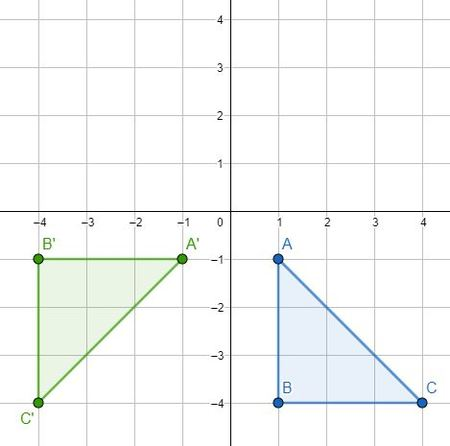270 CCW/90 CW

100

Rotate the point G(4, 3) 180-degrees then translate by the rule (x,y)-->(x+2, y-2) to find G''.

G''(-2,-5)

100

What does it mean to be congruent?

Same shape, same size.

100

What does CPCTC stand for?

Corresponding Parts of Congruent Triangles are Congruent

100

Fill in reason #1.Given

200

Translate the point B(-2, 5) by the rule (x,y)-->(x+2,y)

B'(0,5)

200

What quadrant would W'' be in after reflecting W(2,-6) over the y-axis and then again over the line y=4.

200

List the 5 triangle congruence theorems.

SSS

SAS

ASA

AAS

HL

200

What is the length of VU?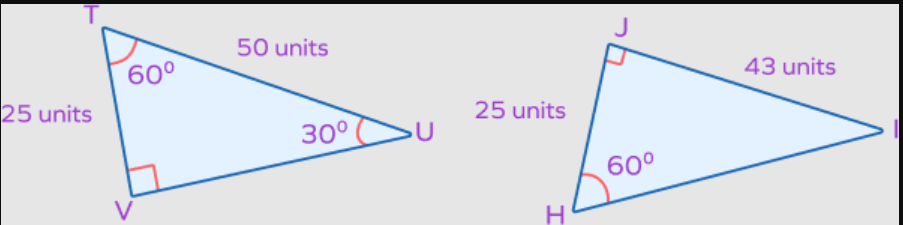43 units

200

Fill in the reason #3.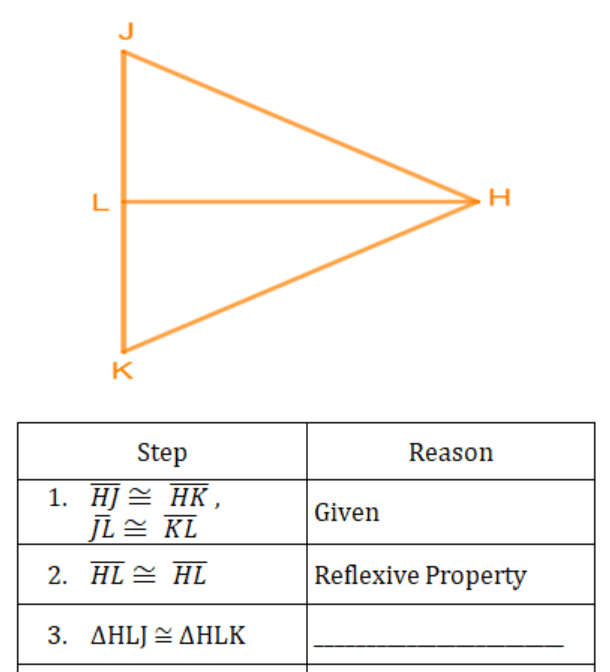SSS

300

Where would C' be after reflecting over the x-axis?C'(3,3)

300

Identify the sequence of transformations that displayed below.Reflect over y-axis then rotate 90-degrees CCW.

300

How are the two triangles congruent?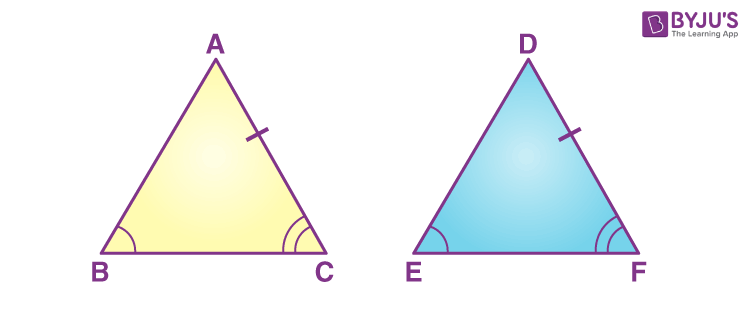AAS

300

The triangles below are congruent via HL. What is y?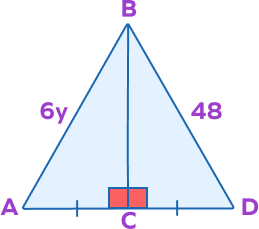y=8
300

Fill in reason #2.Reflexive Property

400

Rotate the point J(42,-37) 90 degrees.

J'(37,42)

400

Identify the sequence of transformations that took UST to U'S'T'.Reflect over x-axis and translate right 5 units.

400

How are the two triangles congruent to each other?HL

400

Solve for x.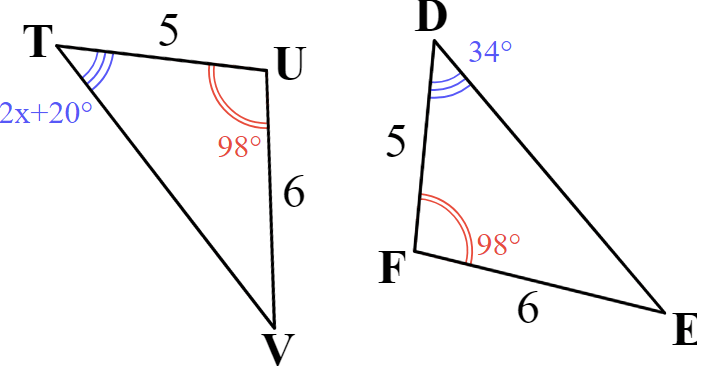x=7

400

Fill in statement #2. Note: F is also the midpoint of DH.EF=GF and DF=HF.
500

Reflect G(-5,-5) over the line y=x.

G'(-5,-5)

500

Find the coordinates of the image of triangle ABC after translating by the rule (x,y)-->(x-2,y-1) then reflecting over the line y=-x.A''(-1,2)

B''(-3,-3)

C''(-1,-3)

500

If

angle1=angle2

, then what more information do you need in order to say the two triangles below are congruent by SAS?MO=TO

500

triangleTUGcongtriangleWAR

If TU=4x+13, WA=6x+7, and GT=3x+11, then how long is WR?

WR=20

500

Fill in reason #2.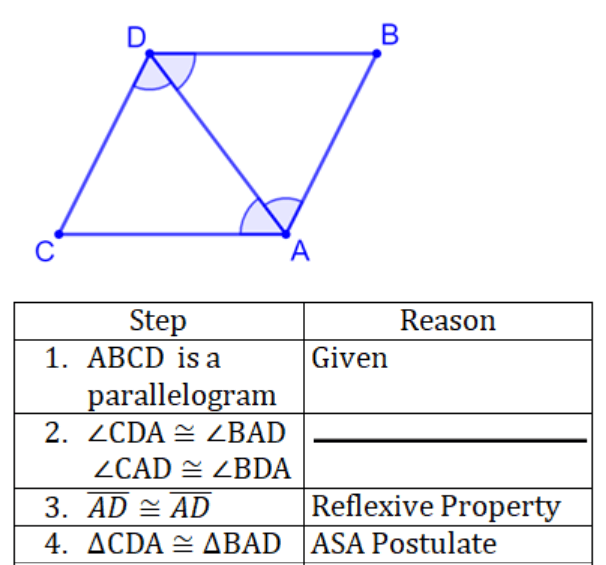Alternate interior angles.

Click to zoom